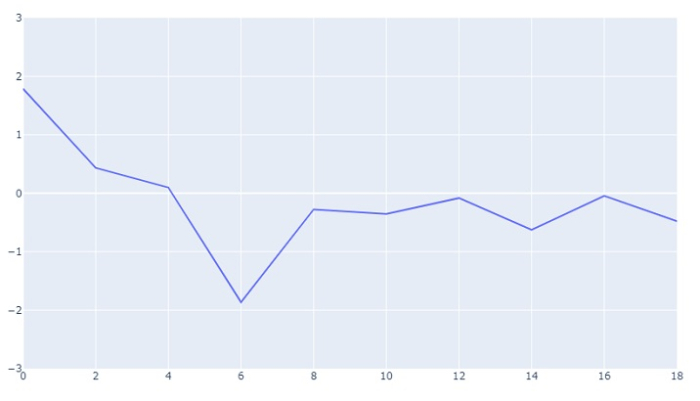# How to set the range of Y-axis in Python Plotly?

Plotly supports to the range on both X and Y axis. Let us understand how to set the range of Y-axis in Plotly.

• plotly.graph_objects is used to generate figures. It contains a lot of methods to customize charts and render a chart in HTML format.

• Create a numpy module and generate random ranges for both X and Y axis.

• Create Figure() method to plot X and Y axis with mode as lines

• Create update_layout() method and set the Y-axis range.

Follow the steps given to set the range of Y-axis in Plotly.

Step 1 − Import plotly

Import plotly.graphs_objs module and alias as go

import plotly.graphs_objs as go

Step 2 − Import numpy

Import numpy module and alias as np and set the random seed value.

import numpy as np
np.random.seed(3)

Step 3 − Generate random number on X-axis

Let us generate a list of random range of numbers on X-axis.

x = list(range(0,20,2))

Step 4 − Generate random number on Y-axis

Generate random number on Y-axis as follows −

y = np.random.randn(10)

Step 5 − Generate the scatter plot

Let us generate the scatter plot with the following coordinates −

fig = go.Figure(data=go.Scatter(x=x, y=y, mode='lines'))

Step 6 − Set the Y-axis range

Set the Y-axis range using update_layout() method.

fig.update_layout(yaxis_range=[-3,3])

Step 7 − Display the figure

Display the figure using show() method.

fig.show()

## Example

The complete code to set the range of Y-axis in Python Plotly is as follows −

# Importing Libraries
import plotly.graph_objs as go
import numpy as np

np.random.seed(3)

# generating numbers ranging from 0 to 18 on X-axis
x = list(range(0,20,2))

# generating random numbers on y-axis
y = np.random.randn(10)

# plotting scatter plot on x and y data with
# 'lines' as mode
fig = go.Figure(data=go.Scatter(x=x, y=y, mode='lines'))

# setting the y-axis range from -3 to 3
fig.update_layout(yaxis_range=[-3,3])

# to display the figure in the output screen
fig.show()

## Output

It will show the following output on the browser −